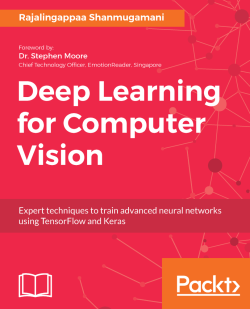# Deep Learning for Computer Vision4.2 (5 reviews total)
By Rajalingappaa Shanmugamani
• \$33.99 eBook
• \$12.99 eBook + Subscription
What do you get with a Packt Subscription?

• Constantly updated with 100+ new titles each month
• Breadth and depth in over 1,000+ technologies

Deep learning has shown its power in several application areas of Artificial Intelligence, especially in Computer Vision. Computer Vision is the science of understanding and manipulating images, and finds enormous applications in the areas of robotics, automation, and so on. This book will also show you, with practical examples, how to develop Computer Vision applications by leveraging the power of deep learning.

In this book, you will learn different techniques related to object classification, object detection, image segmentation, captioning, image generation, face analysis, and more. You will also explore their applications using popular Python libraries such as Tensorflow and Keras. This book will help you master state-of-the-art, deep learning algorithms and their implementation.

Publication date:
January 2018
Publisher
Packt
Pages
310
ISBN
9781788295628

## Chapter 1. Getting Started

Computer vision is the science of understanding or manipulating images and videos. Computer vision has a lot of applications, including autonomous driving, industrial inspection, and augmented reality. The use of deep learning for computer vision can be categorized into multiple categories: classification, detection, segmentation, and generation, both in images and videos. In this book, you will learn how to train deep learning models for computer vision applications and deploy them on multiple platforms. We will use TensorFlow, a popular python library for deep learning throughout this book for the examples. In this chapter, we will cover the following topics:

• The basics and vocabulary of deep learning
• How deep learning meets computer vision?
• Setting up the development environment that will be used for the examples covered in this book
• Getting a feel for TensorFlow, along with its powerful tools, such as TensorBoard and TensorFlow Serving

## Understanding deep learning

Computer vision as a field has a long history. With the emergence of deep learning, computer vision has proven to be useful for various applications. Deep learning is a collection of techniques from artificial neural network (ANN), which is a branch of machine learning. ANNs are modelled on the human brain; there are nodes linked to each other that pass information to each other. In the following sections, we will discuss in detail how deep learning works by understanding the commonly used basic terms.

### Perceptron

An artificial neuron or perceptron takes several inputs and performs a weighted summation to produce an output. The weight of the perceptron is determined during the training process and is based on the training data. The following is a diagram of the perceptron: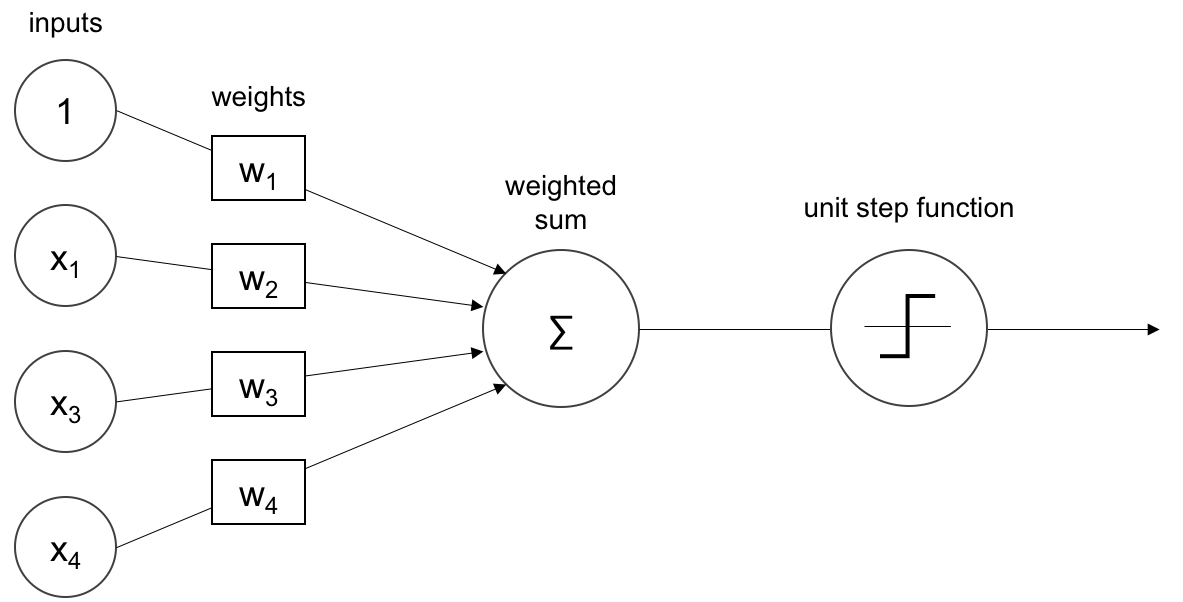The inputs are weighted and summed as shown in the preceding image. The sum is then passed through a unit step function, in this case, for a binary classification problem. A perceptron can only learn simple functions by learning the weights from examples. The process of learning the weights is called training. The training on a perceptron can be done through gradient-based methods which are explained in a later section. The output of the perceptron can be passed through an `activation` function or `transfer` function, which will be explained in the next section.

### Activation functions

The `activation` functions make neural nets nonlinear. An activation function decides whether a perceptron should fire or not. During training activation, functions play an important role in adjusting the gradients. An `activation` function such as sigmoid, shown in the next section, attenuates the values with higher magnitudes. This nonlinear behaviour of the `activation` function gives the deep nets to learn complex functions. Most of the `activation` functions are continuous and differential functions, except rectified unit at 0. A continuous function has small changes in output for every small change in input. A differential function has a derivative existing at every point in the domain.

In order to train a neural network, the function has to be differentiable. Following are a few `activation` functions.

### Note

Don't worry if you don't understand the terms like continuous and differentiable in detail. It will become clearer over the chapters.

#### Sigmoid

Sigmoid can be considered a smoothened step function and hence differentiable. Sigmoid is useful for converting any value to probabilities and can be used for binary classification. The sigmoid maps input to a value in the range of 0 to 1, as shown in the following graph: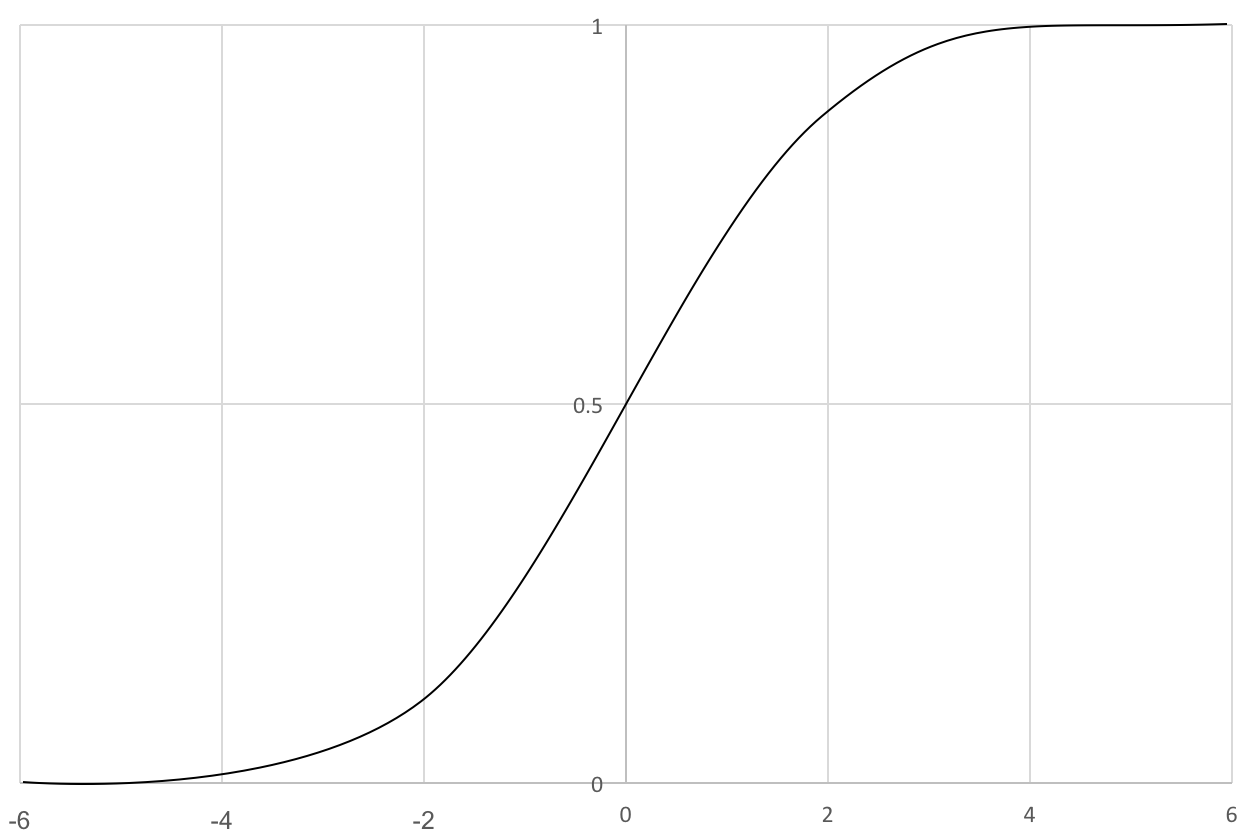The change in Y values with respect to X is going to be small, and hence, there will be vanishing gradients. After some learning, the change may be small. Another activation function called `tanh`, explained in next section, is a scaled version of sigmoid and avoids the problem of a vanishing gradient.

#### The hyperbolic tangent function

The hyperbolic tangent function, or `tanh`, is the scaled version of sigmoid. Like sigmoid, it is smooth and differentiable. The `tanh` maps input to a value in the range of -1 to 1, as shown in the following graph: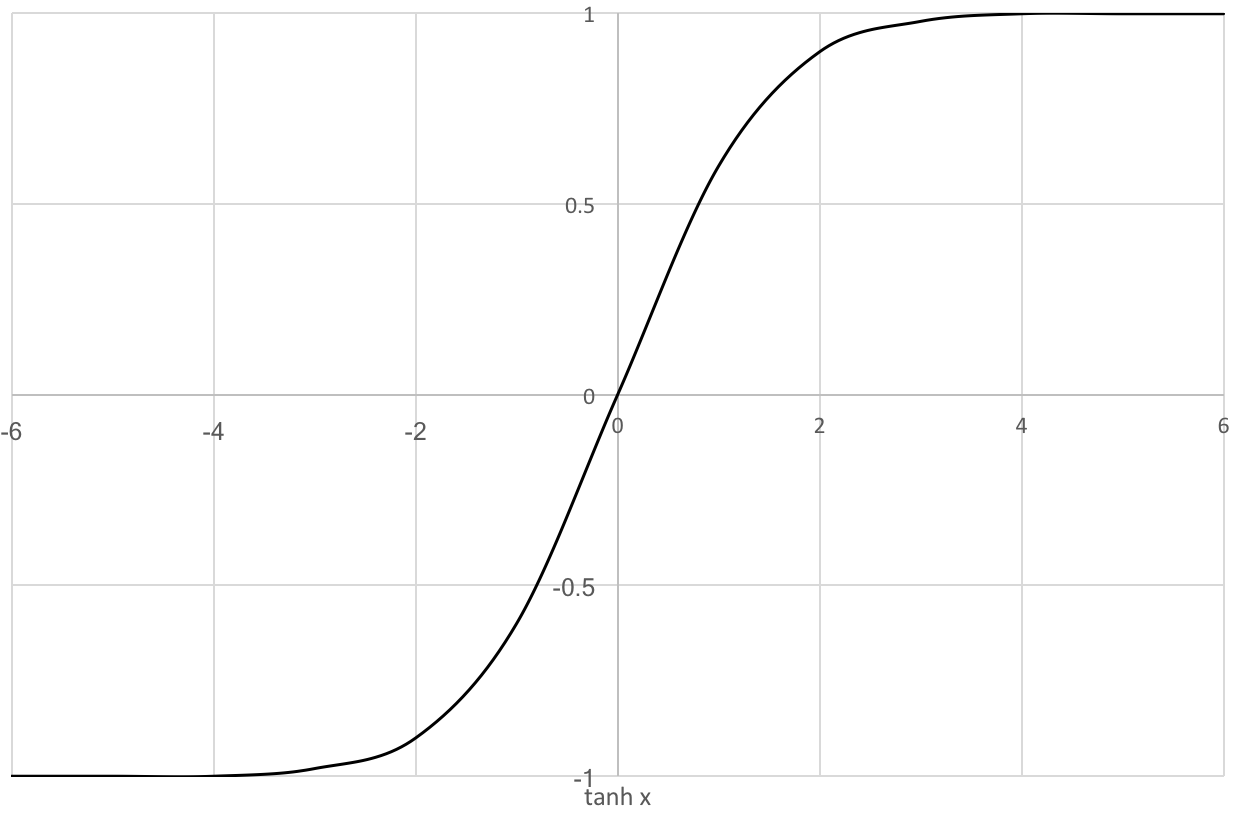The gradients are more stable than sigmoid and hence have fewer vanishing gradient problems. Both sigmoid and `tanh` fire all the time, making the ANN really heavy. The Rectified Linear Unit (ReLU) activation function, explained in the next section, avoids this pitfall by not firing at times.

#### The Rectified Linear Unit (ReLU)

ReLu can let big numbers pass through. This makes a few neurons stale and they don't fire. This increases the sparsity, and hence, it is good. The `ReLU` maps input x to max (0, x), that is, they map negative inputs to 0, and positive inputs are output without any change as shown in the following graph: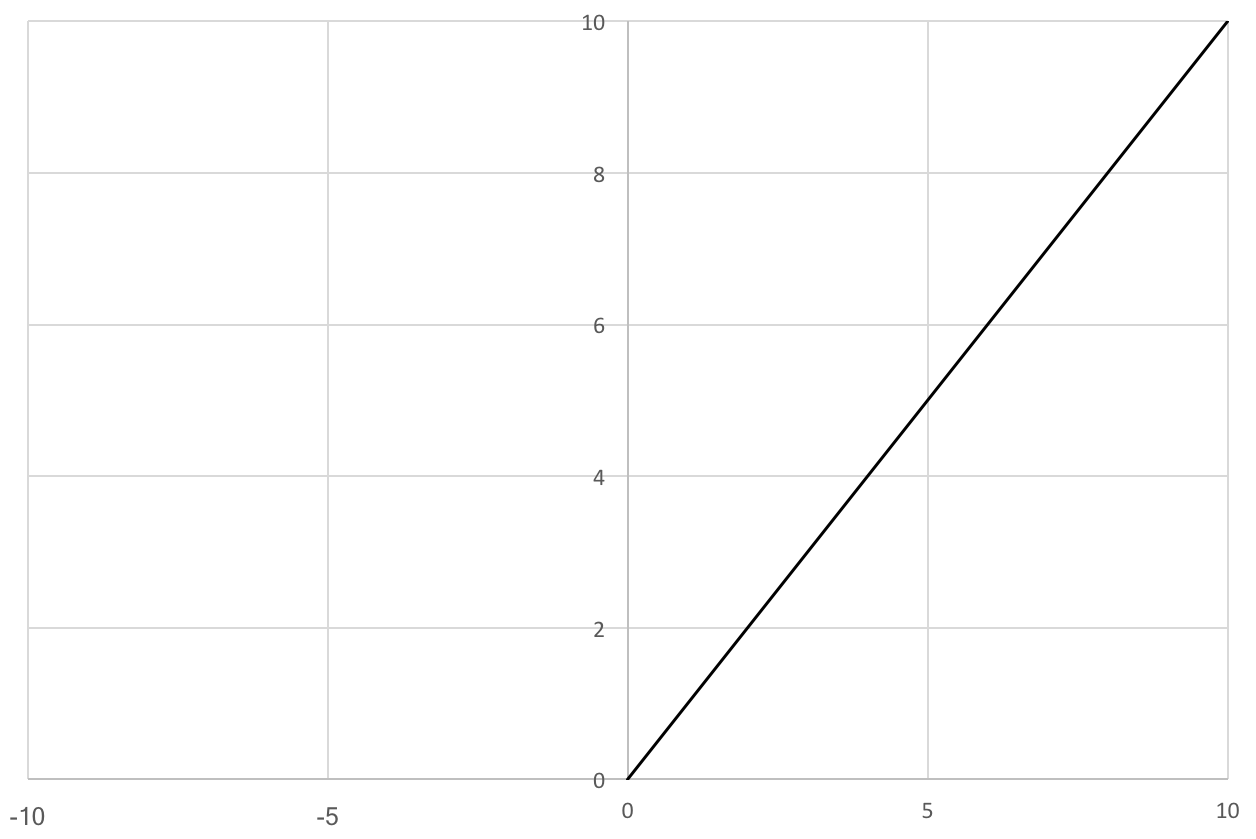Because ReLU doesn't fire all the time, it can be trained faster. Since the function is simple, it is computationally the least expensive. Choosing the `activation` function is very dependent on the application. Nevertheless, ReLU works well for a large range of problems. In the next section, you will learn how to stack several perceptrons together that can learn more complex functions than perceptron.

### Artificial neural network (ANN)

ANN is a collection of perceptrons and `activation` functions. The perceptrons are connected to form hidden layers or units. The hidden units form the nonlinear basis that maps the input layers to output layers in a lower-dimensional space, which is also called artificial neural networks. ANN is a map from input to output. The map is computed by weighted addition of the inputs with biases. The values of weight and bias values along with the architecture are called `model`.

The training process determines the values of these weights and biases. The model values are initialized with random values during the beginning of the training. The error is computed using a loss function by contrasting it with the ground truth. Based on the loss computed, the weights are tuned at every step. The training is stopped when the error cannot be further reduced. The training process learns the features during the training. The features are a better representation than the raw images. The following is a diagram of an artificial neural network, or multi-layer perceptron: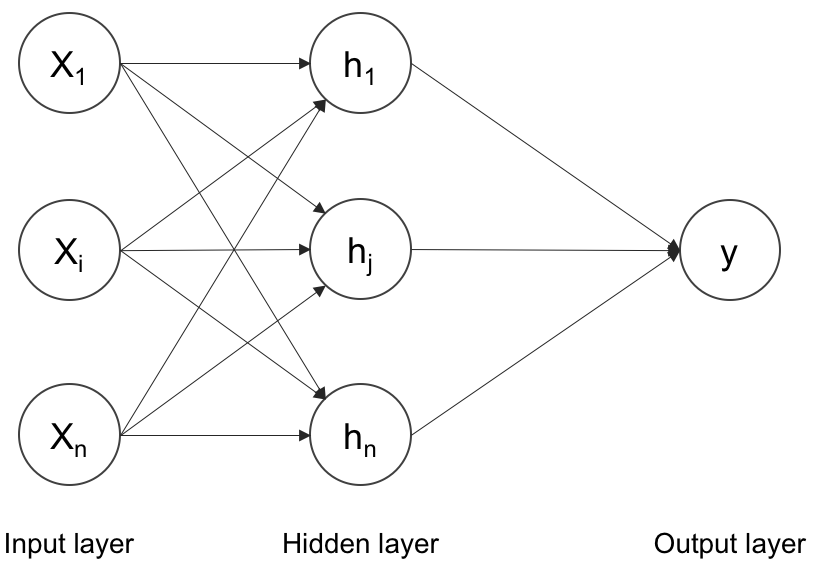Several inputs of x are passed through a hidden layer of perceptrons and summed to the output. The universal approximation theorem suggests that such a neural network can approximate any function. The hidden layer can also be called a dense layer. Every layer can have one of the `activation` functions described in the previous section. The number of hidden layers and perceptrons can be chosen based on the problem. There are a few more things that make this multilayer perceptron work for multi-class classification problems. A multi-class classification problem tries to discriminate more than ten categories. We will explore those terms in the following sections.

#### One-hot encoding

One-hot encoding is a way to represent the target variables or classes in case of a classification problem. The target variables can be converted from the string labels to one-hot encoded vectors. A one-hot vector is filled with 1 at the index of the target class but with 0 everywhere else. For example, if the target classes are cat and dog, they can be represented by [1, 0] and [0, 1], respectively. For 1,000 classes, one-hot vectors will be of size 1,000 integers with all zeros but 1. It makes no assumptions about the similarity of target variables. With the combination of one-hot encoding with softmax explained in the following section, multi-class classification becomes possible in ANN.

#### Softmax

Softmax is a way of forcing the neural networks to output the sum of 1. Thereby, the output values of the `softmax` function can be considered as part of a probability distribution. This is useful in multi-class classification problems. Softmax is a kind of `activation` function with the speciality of output summing to 1. It converts the outputs to probabilities by dividing the output by summation of all the other values. The Euclidean distance can be computed between softmax probabilities and one-hot encoding for optimization. But the cross-entropy explained in the next section is a better cost function to optimize.

#### Cross-entropy

Cross-entropy compares the distance between the outputs of softmax and one-hot encoding. Cross-entropy is a loss function for which error has to be minimized. Neural networks estimate the probability of the given data to every class. The probability has to be maximized to the correct target label. Cross-entropy is the summation of negative logarithmic probabilities. Logarithmic value is used for numerical stability. Maximizing a function is equivalent to minimizing the negative of the same function. In the next section, we will see the following regularization methods to avoid the overfitting of ANN:

• Dropout
• Batch normalization
• L1 and L2 normalization

#### Dropout

Dropout is an effective way of regularizing neural networks to avoid the overfitting of ANN. During training, the dropout layer cripples the neural network by removing hidden units stochastically as shown in the following image: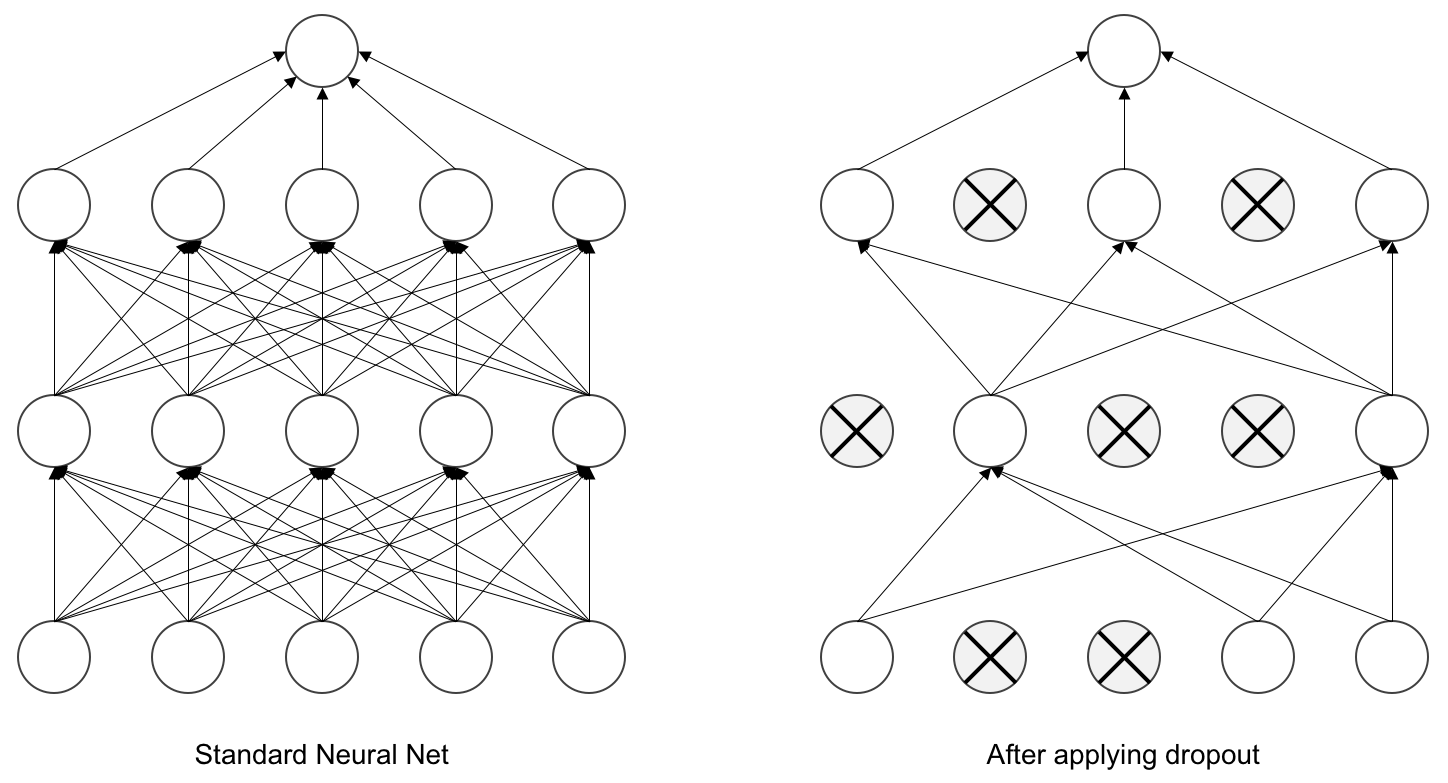Note how the neurons are randomly trained. Dropout is also an efficient way of combining several neural networks. For each training case, we randomly select a few hidden units so that we end up with different architectures for each case. This is an extreme case of bagging and model averaging. Dropout layer should not be used during the inference as it is not necessary.

#### Batch normalization

Batch normalization, or batch-norm, increase the stability and performance of neural network training. It normalizes the output from a layer with zero mean and a standard deviation of 1. This reduces overfitting and makes the network train faster. It is very useful in training complex neural networks.

#### L1 and L2 regularization

L1 penalizes the absolute value of the weight and tends to make the weights zero. L2 penalizes the squared value of the weight and tends to make the weight smaller during the training. Both the regularizes assume that models with smaller weights are better.

### Training neural networks

Training ANN is tricky as it contains several parameters to optimize. The procedure of updating the weights is called backpropagation. The procedure to minimize the error is called optimization. We will cover both of them in detail in the next sections.

#### Backpropagation

A backpropagation algorithm is commonly used for training artificial neural networks. The weights are updated from backward based on the error calculated as shown in the following image: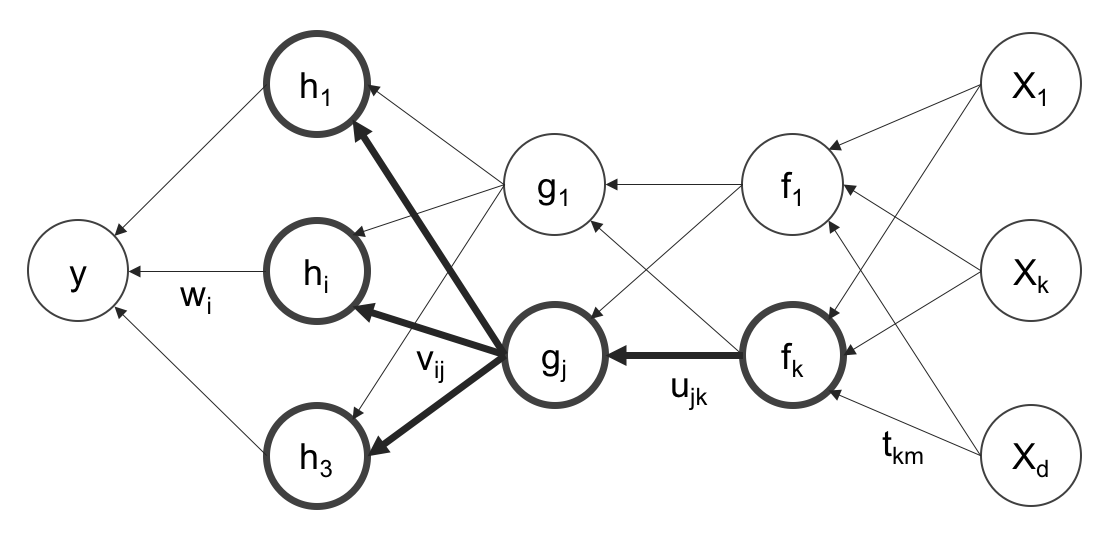After calculating the error, gradient descent can be used to calculate the weight updating, as explained in the next section.

The gradient descent algorithm performs multidimensional optimization. The objective is to reach the global maximum. Gradient descent is a popular optimization technique used in many machine-learning models. It is used to improve or optimize the model prediction. One implementation of gradient descent is called the stochastic gradient descent (SGD) and is becoming more popular (explained in the next section) in neural networks. Optimization involves calculating the error value and changing the weights to achieve that minimal error. The direction of finding the minimum is the negative of the gradient of the `loss` function. The gradient descent procedure is qualitatively shown in the following figure: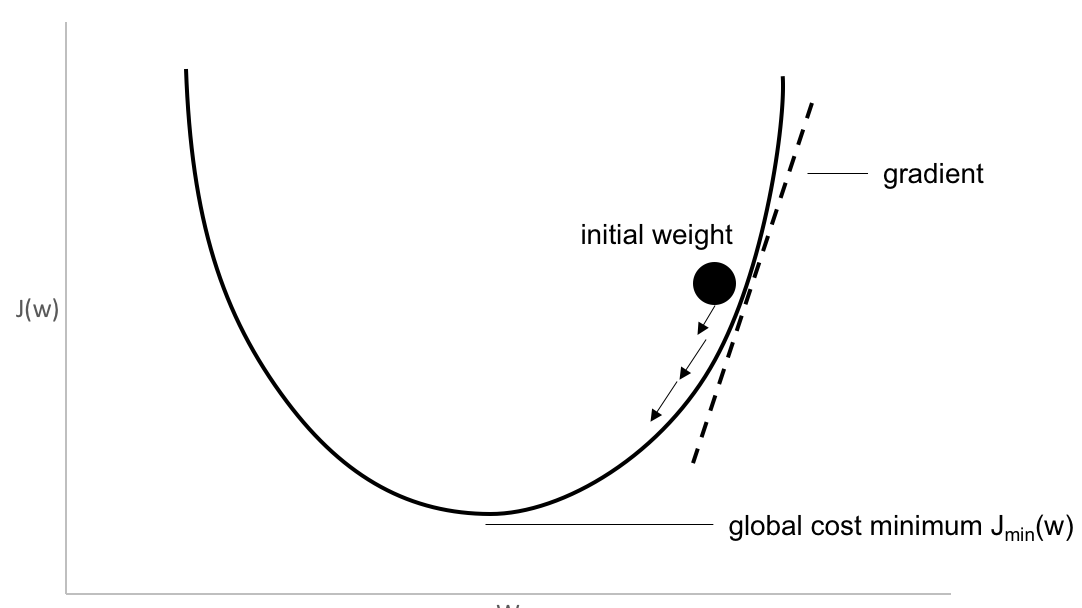The learning rate determines how big each step should be. Note that the ANN with nonlinear activations will have local minima. SGD works better in practice for optimizing non-convex cost functions.

SGD is the same as gradient descent, except that it is used for only partial data to train every time. The parameter is called mini-batch size. Theoretically, even one example can be used for training. In practice, it is better to experiment with various numbers. In the next section, we will discuss convolutional neural networks that work better on image data than the standard ANN.

### Note

Visit https://yihui.name/animation/example/grad-desc/ to see a great visualization of gradient descent on convex and non-convex surfaces.

### Playing with TensorFlow playground

TensorFlow playground is an interactive visualization of neural networks. Visit http://playground.tensorflow.org/, play by changing the parameters to see how the previously mentioned terms work together. Here is a screenshot of the playground: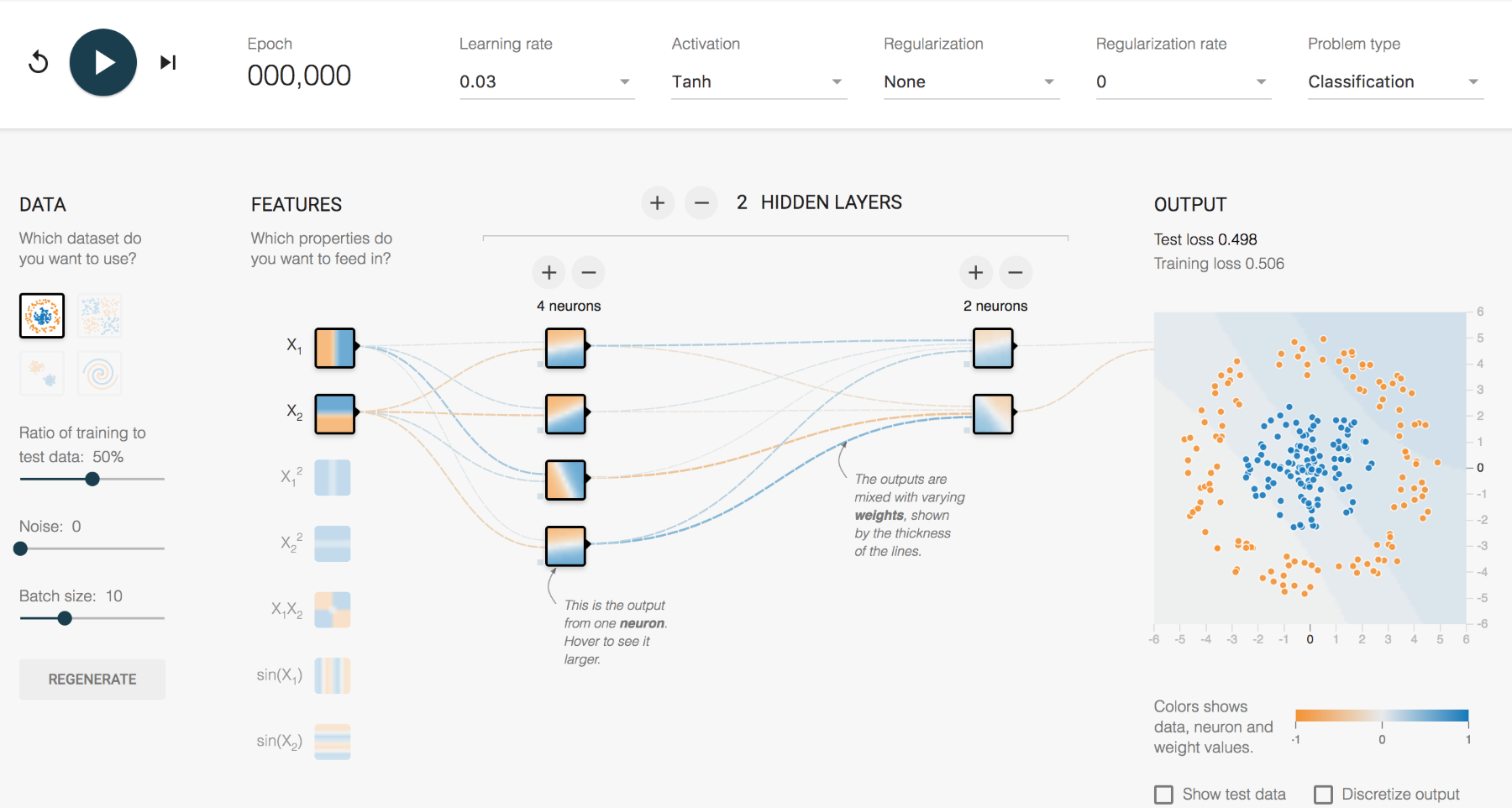Dashboard in the TensorFlow playground

As shown previously, the reader can change learning rate, activation, regularization, hidden units, and layers to see how it affects the training process. You can spend some time adjusting the parameters to get the intuition of how neural networks for various kinds of data.

### Convolutional neural network

Convolutional neural networks (CNN) are similar to the neural networks described in the previous sections. CNNs have weights, biases, and outputs through a nonlinear activation. Regular neural networks take inputs and the neurons fully connected to the next layers. Neurons within the same layer don't share any connections. If we use regular neural networks for images, they will be very large in size due to a huge number of neurons, resulting in overfitting. We cannot use this for images, as images are large in size. Increase the model size as it requires a huge number of neurons. An image can be considered a volume with dimensions of height, width, and depth. Depth is the channel of an image, which is red, blue, and green. The neurons of a CNN are arranged in a volumetric fashion to take advantage of the volume. Each of the layers transforms the input volume to an output volume as shown in the following image: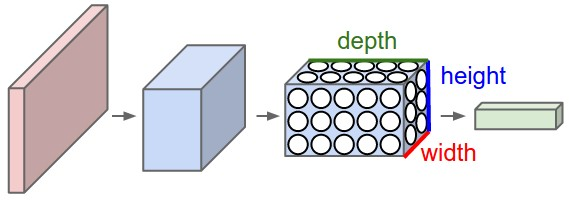Convolution neural network filters encode by transformation. The learned filters detect features or patterns in images. The deeper the layer, the more abstract the pattern is. Some analyses have shown that these layers have the ability to detect edges, corners, and patterns. The learnable parameters in CNN layers are less than the dense layer described in the previous section.

#### Kernel

Kernel is the parameter convolution layer used to convolve the image. The convolution operation is shown in the following figure: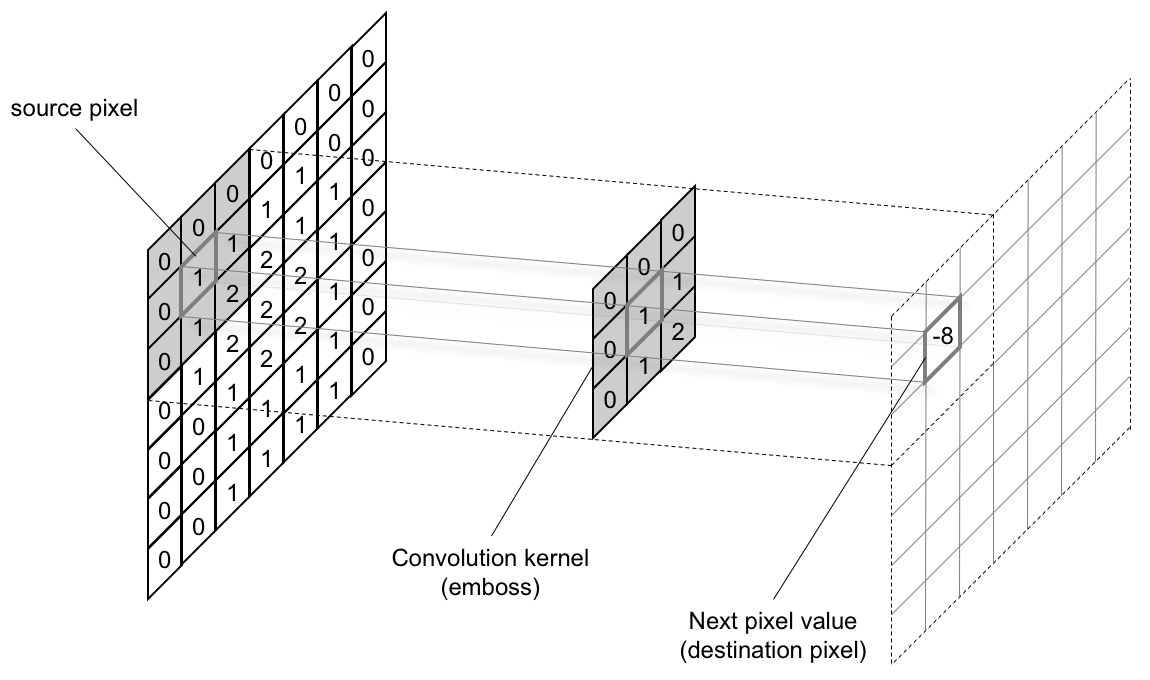The kernel has two parameters, called stride and size. The size can be any dimension of a rectangle. Stride is the number of pixels moved every time. A stride of length 1 produces an image of almost the same size, and a stride of length 2 produces half the size. Padding the image will help in achieving the same size of the input.

#### Max pooling

Pooling layers are placed between convolution layers. Pooling layers reduce the size of the image across layers by sampling. The sampling is done by selecting the maximum value in a window. Average pooling averages over the window. Pooling also acts as a regularization technique to avoid overfitting. Pooling is carried out on all the channels of features. Pooling can also be performed with various strides.

The size of the window is a measure of the receptive field of CNN. The following figure shows an example of max pooling: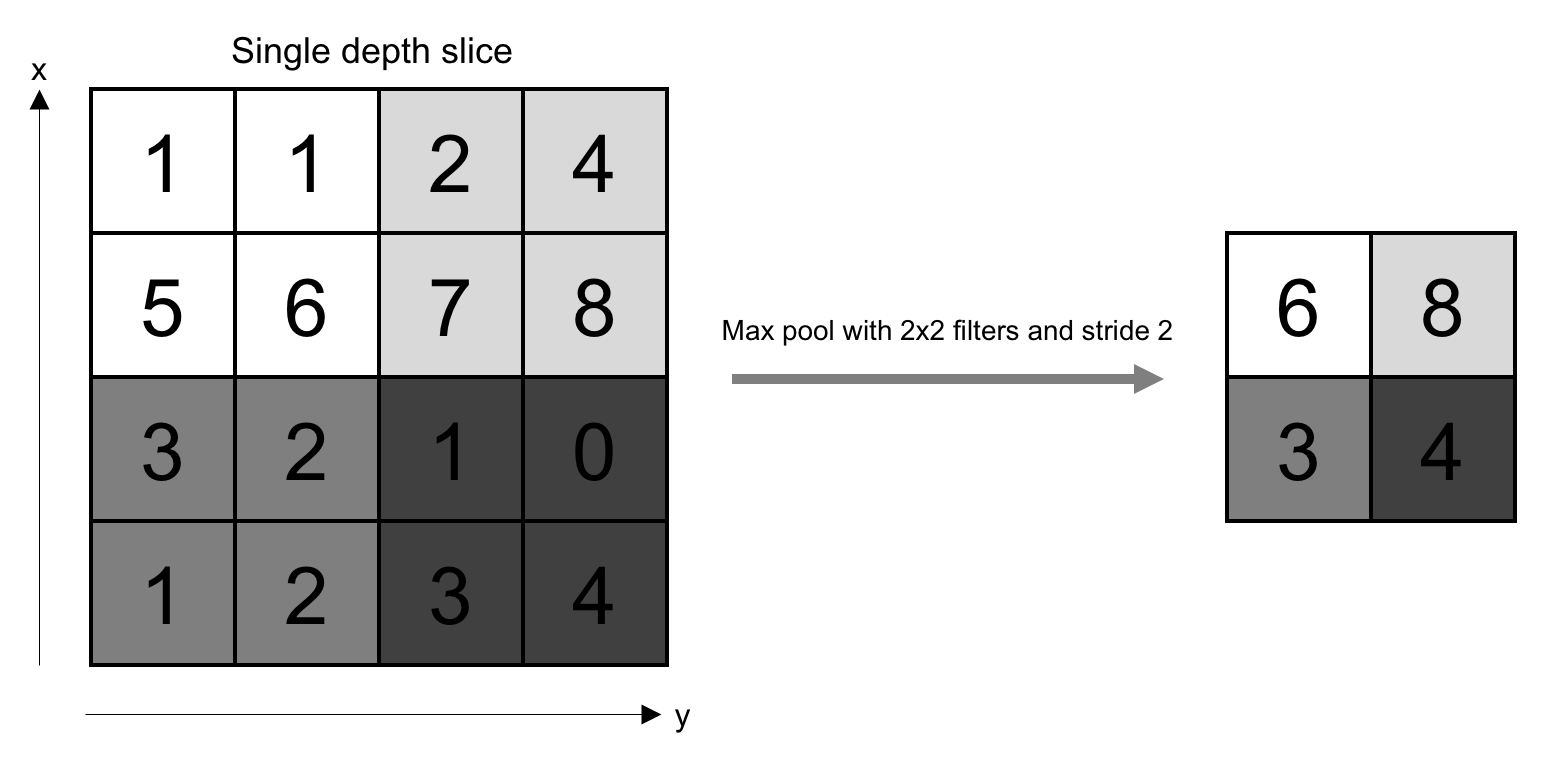CNN is the single most important component of any deep learning model for computer vision. It won't be an exaggeration to state that it will be impossible for any computer to have vision without a CNN. In the next sections, we will discuss a couple of advanced layers that can be used for a few applications.

### Note

Visit https://www.youtube.com/watch?v=jajksuQW4mc for a great visualization of a CNN and max-pooling operation.

### Recurrent neural networks (RNN)

Recurrent neural networks (RNN) can model sequential information. They do not assume that the data points are intensive. They perform the same task from the output of the previous data of a series of sequence data. This can also be thought of as memory. RNN cannot remember from longer sequences or time. It is unfolded during the training process, as shown in the following image: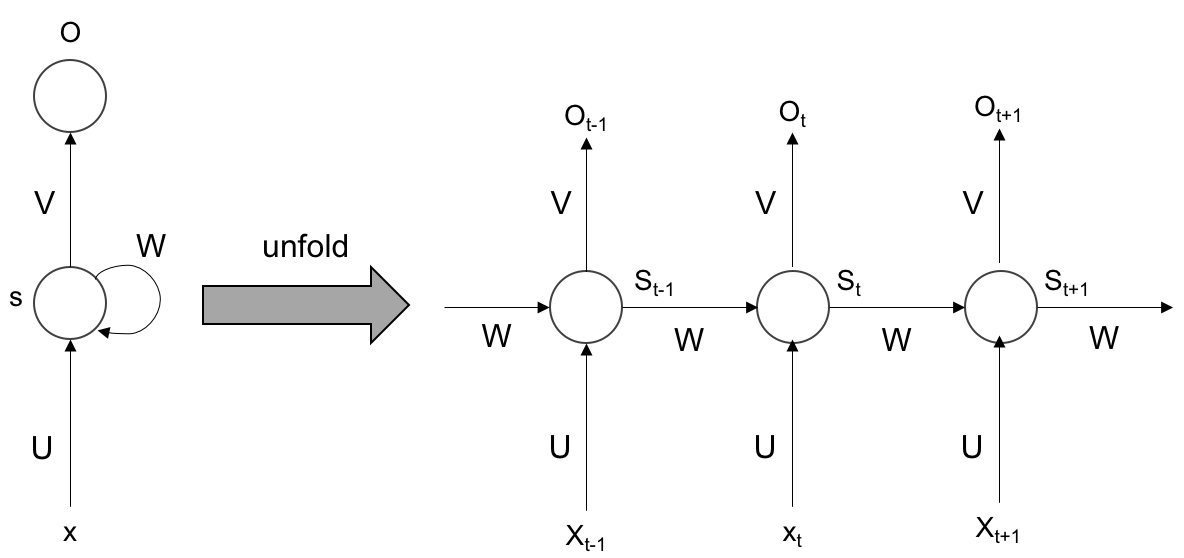As shown in the preceding figure, the step is unfolded and trained each time. During backpropagation, the gradients can vanish over time. To overcome this problem, Long short-term memory can be used to remember over a longer time period.

### Long short-term memory (LSTM)

Long short-term memory (LSTM) can store information for longer periods of time, and hence, it is efficient in capturing long-term efficiencies. The following figure illustrates how an LSTM cell is designed: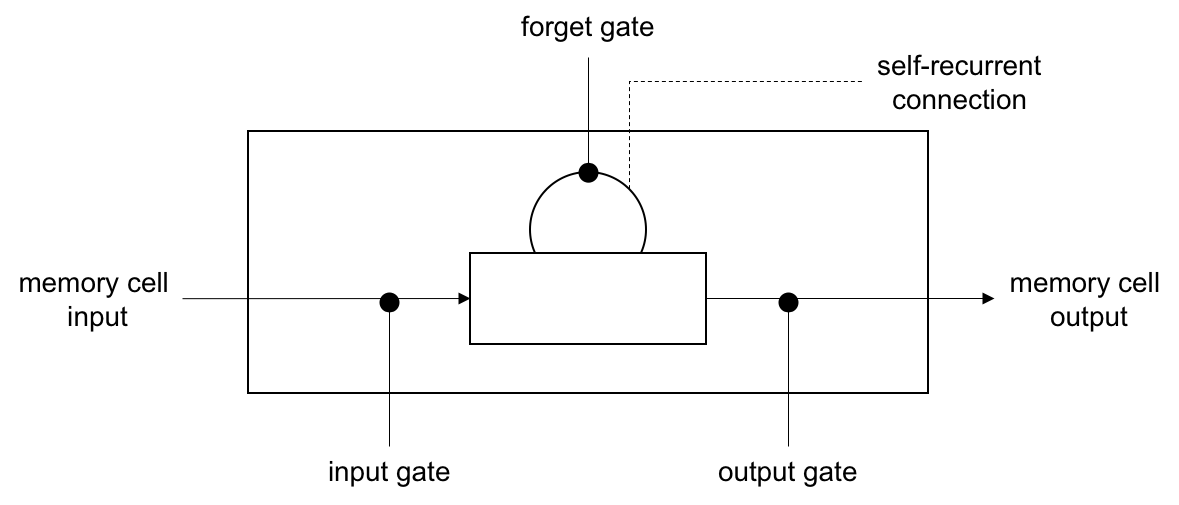LSTM has several gates: forget, input, and output. Forget gate maintains the information previous state. The input gate updates the current state using the input. The output gate decides the information be passed to the next state. The ability to forget and retain only the important things enables LSTM to remember over a longer time period. You have learned the deep learning vocabulary that will be used throughout the book. In the next section, we will see how deep learning can be used in the context of computer vision.

## Deep learning for computer vision

Computer vision enables the properties of human vision on a computer. A computer could be in the form of a smartphone, drones, CCTV, MRI scanner, and so on, with various sensors for perception. The sensor produces images in a digital form that has to be interpreted by the computer. The basic building block of such interpretation or intelligence is explained in the next section. The different problems that arise in computer vision can be effectively solved using deep learning techniques.

### Classification

Image classification is the task of labelling the whole image with an object or concept with confidence. The applications include gender classification given an image of a person's face, identifying the type of pet, tagging photos, and so on. The following is an output of such a classification task: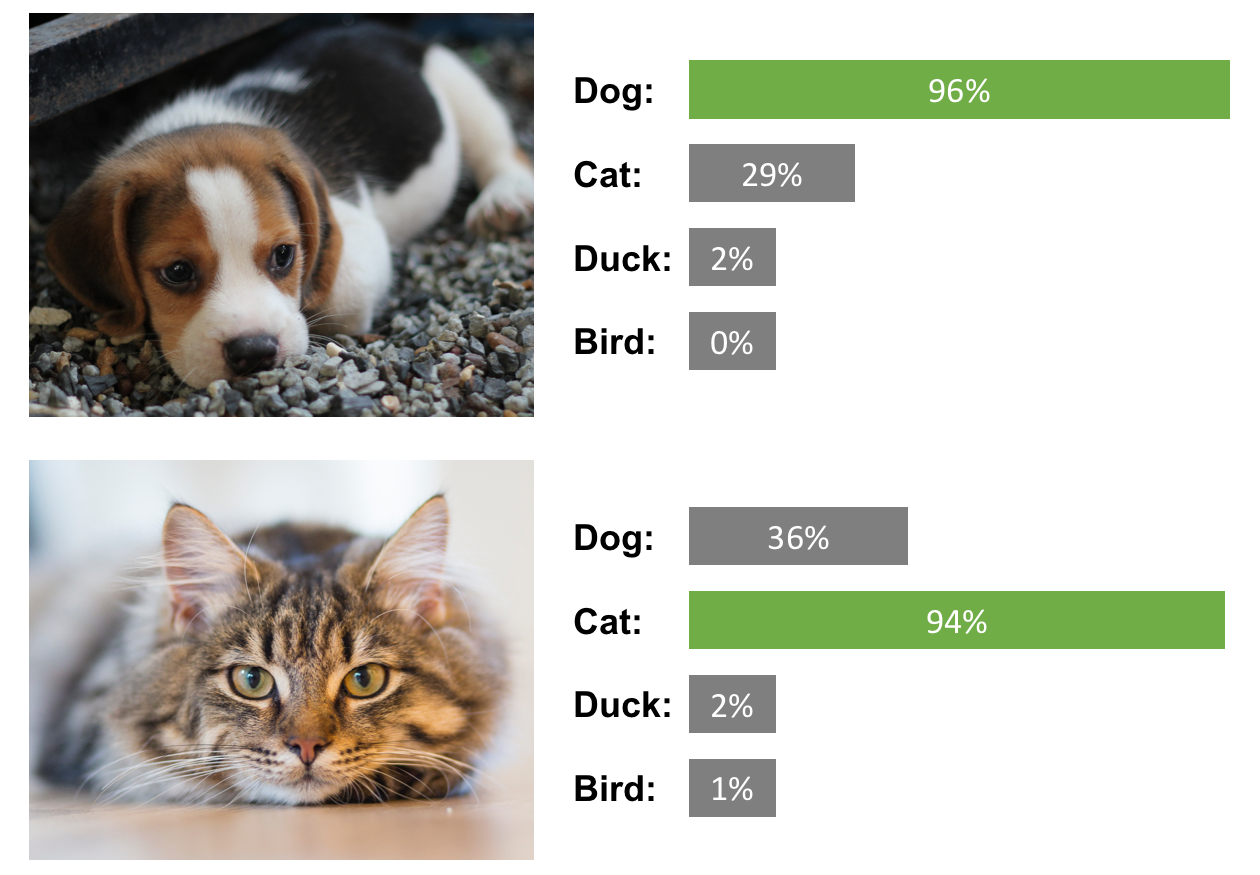The Chapter 2, Image Classification, covers in detail the methods that can be used for classification tasks and in Chapter 3, Image Retrieval, we use the classification models for visualization of deep learning models and retrieve similar images.

### Detection or localization and segmentation

Detection or localization is a task that finds an object in an image and localizes the object with a bounding box. This task has many applications, such as finding pedestrians and signboards for self-driving vehicles. The following image is an illustration of detection: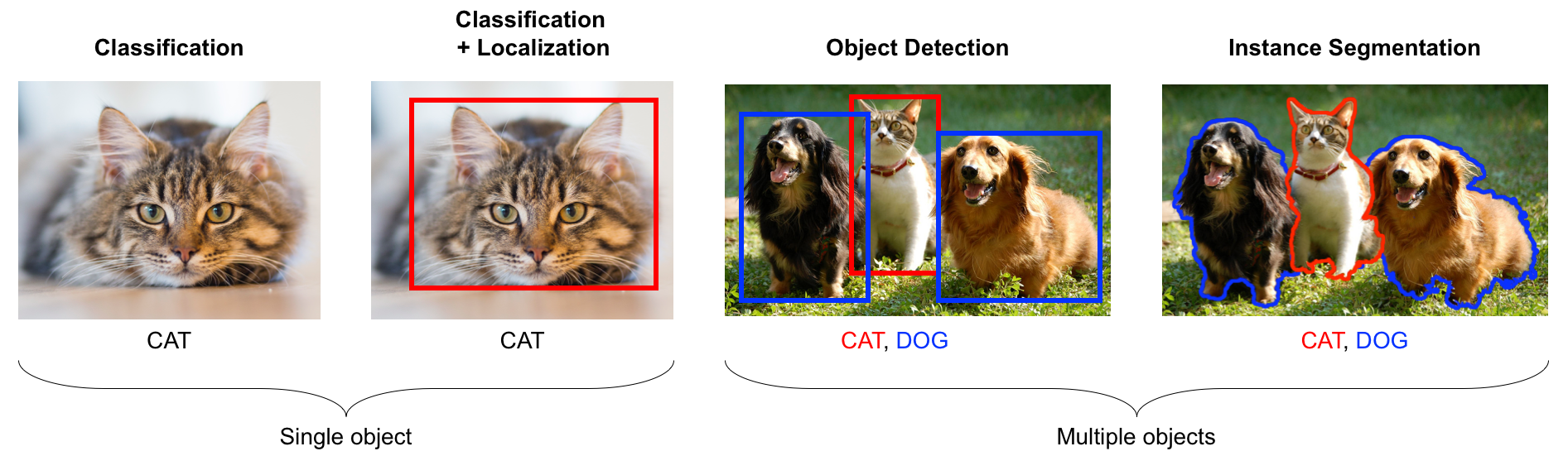Segmentation is the task of doing pixel-wise classification. This gives a fine separation of objects. It is useful for processing medical images and satellite imagery. More examples and explanations can be found in Chapter 4, Object Detection and Chapter 5, Image Segmentation.

### Similarity learning

Similarity learning is the process of learning how two images are similar. A score can be computed between two images based on the semantic meaning as shown in the following image: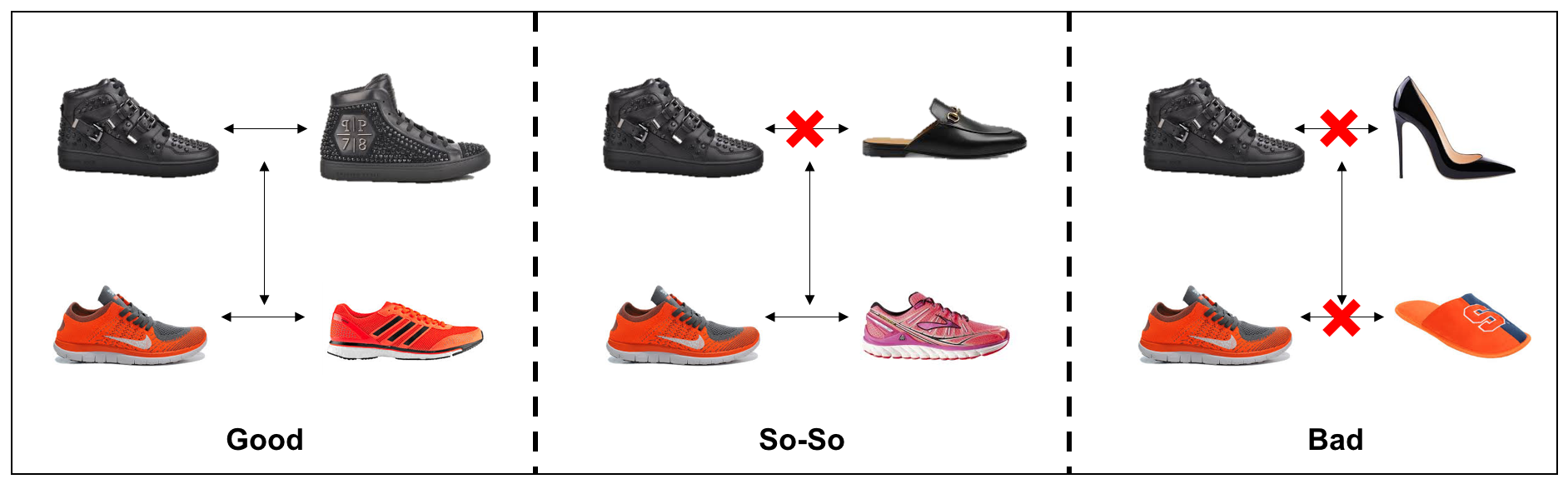There are several applications of this, from finding similar products to performing the facial identification. Chapter 6, Similarity learning, deals with similarity learning techniques.

### Image captioning

Image captioning is the task of describing the image with text as shown [below] here: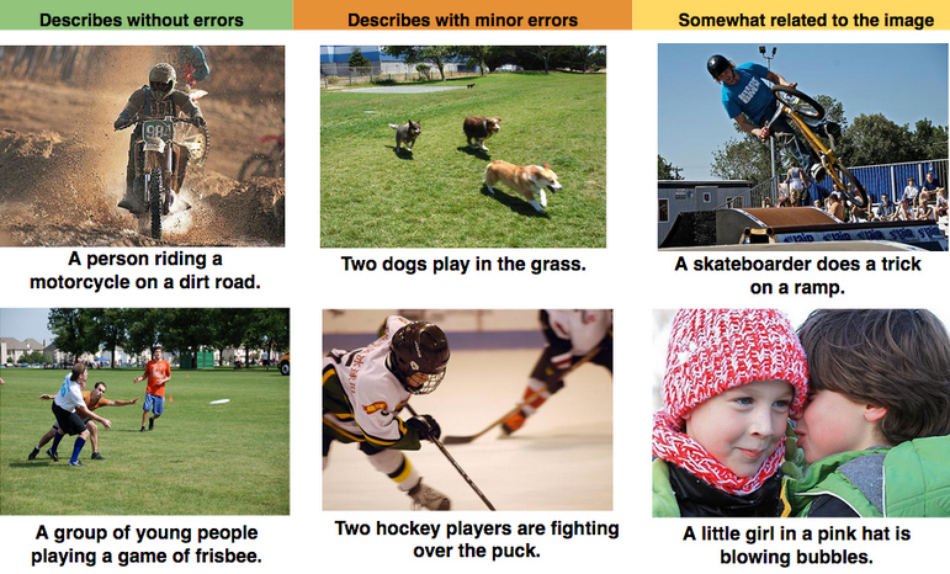Reproduced with permission from Vinyals et al.

The Chapter 8, Image Captioning, goes into detail about image captioning. This is a unique case where techniques of natural language processing (NLP) and computer vision have to be combined.

### Generative models

Generative models are very interesting as they generate images. The following is an example of style transfer application where an image is generated with the content of that image and style of other images: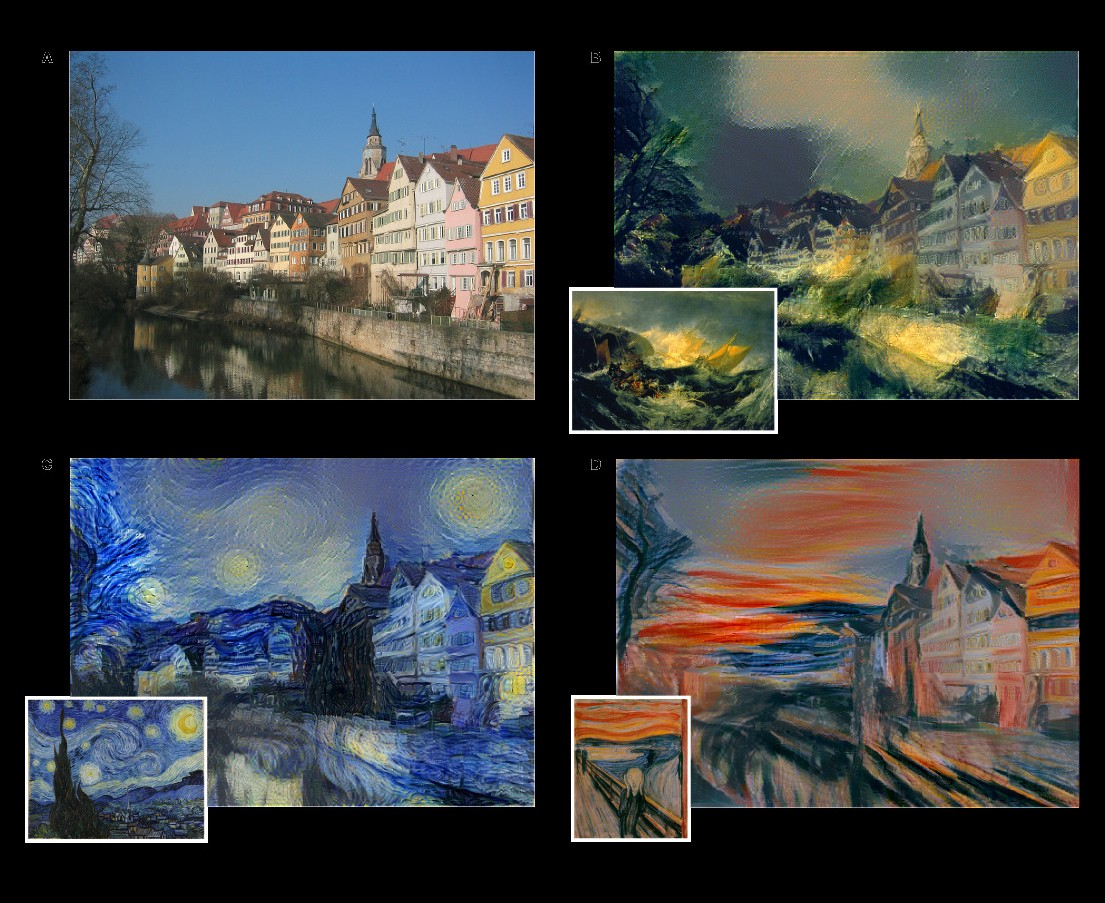Reproduced with permission from Gatys et al.

Images can be generated for other purposes such as new training examples, super-resolution images, and so on. The Chapter 7, Generative Models, goes into detail of generative models.

### Video analysis

Video analysis processes a video as a whole, as opposed to images as in previous cases. It has several applications, such as sports tracking, intrusion detection, and surveillance cameras. Chapter 9, Video Classification, deals with video-specific applications. The new dimension of temporal data gives rise to lots of interesting applications. In the next section, we will see how to set up the development environment.

## Development environment setup

In this section, we will set up the programming environment that will be useful for following the examples in the rest of the book. Readers may have the following choices of Operating Systems:

• Development Operating Systems(OS) such as Mac, Ubuntu, or Windows
• Deployment Operating Systems such as Mac, Windows, Android, iOs, or Ubuntu installed in Cloud platform such as Amazon Web Services (AWS), Google Cloud Platform (GCP), Azure, Tegra, Raspberry Pi

Irrespective of the platforms, all the code developed in this book should run without any issues. In this chapter, we will cover the installation procedures for the development environment. In Chapter 10, Deployment, we will cover installation for deployment in various other environments, such as AWS, GCP, Azure, Tegra, and Raspberry Pi.

### Hardware and Operating Systems - OS

For the development environment, you need to have a lot of computing power as training is significantly computationally expensive. Mac users are rather limited to computing power. Windows and Ubuntu users can beef up their development environment with more processors and General Purpose - Graphics Processing Unit (GP-GPU), which will be explained in the next section.

#### General Purpose - Graphics Processing Unit (GP-GPU)

GP-GPUs are special hardware that speeds up the training process of training deep learning models. The GP-GPUs supplied by NVIDIA company are very popular for deep learning training and deployment as it has well-matured software and community support. Readers can set up a machine with such a GP-GPU for faster training. There are plenty of choices available, and the reader can choose one based on budget. It is also important to choose the RAM, CPU, and hard disk corresponding to the power of the GP-GPU. After the installation of the hardware, the following drivers and libraries have to be installed. Readers who are using Mac, or using Windows/Ubuntu without a GP-GPU, can skip the installation.

The following are the libraries that are required for setting up the environment:

• Computer Unified Device Architecture (CUDA)
• CUDA Deep Neural Network (CUDNN)
##### Computer Unified Device Architecture - CUDA

CUDA is the API layer provided by NVIDIA, using the parallel nature of the GPU. When this is installed, drivers for the hardware are also installed. First, download the `CUDA` library from the NVIDIA-portal: https://developer.nvidia.com/cuda-downloads.

Go through the instructions on the page, download the driver, and follow the installation instructions. Here is the screenshot of Ubuntu CUDA and the installation instructions: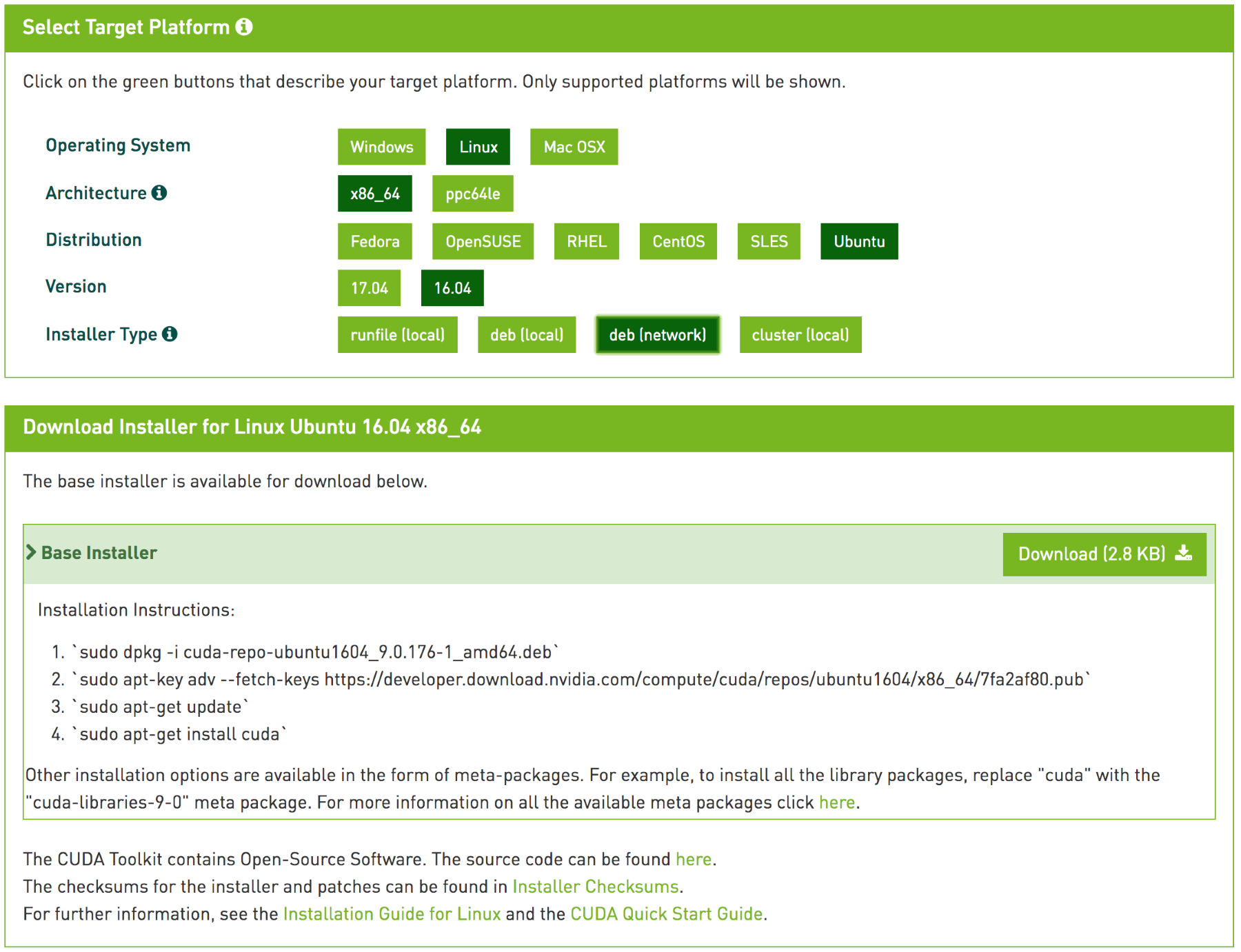These commands would have installed the `cuda-drivers` and the other CUDA APIs required.

### Note

You can check whether the drivers are properly installed by typing `nvidia-smi` in the command prompt.

##### CUDA Deep Neural Network - CUDNN

The `CUDNN` library provides primitives for deep learning algorithms. Since this package is provided by NVIDIA, it is highly optimized for their hardware and runs faster. Several standard routines for deep learning are provided in this package. These packages are used by famous deep learning libraries such as `tensorflow`, `caffe`, and so on. In the next section, instructions are provided for installing `CUDNN`. You can download `CUDNN` from the NVIDIA portal at https://developer.nvidia.com/rdp/cudnn-download.

### Note

User account is required (free signup).

Copy the relevant files to the `CUDA` folders, making them faster to run on GPUs. We will not use `CUDA` and `CUDNN` libraries directly. Tensorflow uses these to work on GP-GPU with optimized routines.

### Installing software packages

There are several libraries required for trained deep learning models. We will install the following libraries and see the reason for selecting the following packages over the competing packages:

• Python and other dependencies
• OpenCV
• TensorFlow
• Keras

#### Python

Python is the de-facto choice for any data science application. It has the largest community and support ecosystem of libraries. TensorFlow API for Python is the most complete, and hence, Python is the natural language of choice. Python has two versions—Python2.x and Python3.x. In this book, we will discuss Python3.x. There are several reasons for this choice:

• Python 2.x development will be stopped by 2020, and hence, Python3.x is the future of Python
• Python 3.x avoids many design flaws in the original implementation
• Contrary to popular belief, Python3.x has as many supporting libraries for data science as Python 2.x.

We will use Python version 3 throughout this book. Go to https://www.python.org/downloads/ and download version 3 according to the OS. Install Python by following the steps given in the download link. After installing Python, pip3 has to be installed for easy installation of Python packages. Then install the several Python packages by entering the following command, so that you can install `OpenCV` and `tensorflow` later:

``` sudo pip3 install numpy scipyscikit-learnpillowh5py
```

The description of the preceding installed packages is given as follows:

• `numpy` is a highly-optimized numerical computation package. It has a powerful N-dimensional package array object, and the matrix operations of `numpy` library are highly optimized for speed. An image can be stored as a 3-dimensional `numpy` object.
• `scipy` has several routines for scientific and engineering calculations. We will use some optimization packages later in the book.
• `scikit-learn` is a machine-learning library from which we will use many helper functions.
• `Ppillow` is useful for image loading and basic operations.
• `H5py` package is a Pythonic interface to the HDF5 binary data format. This is the format to store models trained using Keras.

#### Open Computer Vision - OpenCV

The `OpenCV` is a famous computer vision library. There are several image processing routines available in this library that can be of great use. Following is the step of installing OpenCV in Ubuntu.

`sudo apt-get install python-opencv`

Similar steps can be found for other OSes at https://opencv.org/. It is cross-platform and optimized for CPU-intensive applications. It has interfaces for several programming languages and is supported by Windows, Ubuntu, and Mac.

#### The TensorFlow library

The `tensorflow` is an open source library for the development and deployment of deep learning models. TensorFlow uses computational graphs for data flow and numerical computations. In other words, data, or tensor, flows through the graph, thus the name `tensorflow`. The graph has nodes that enable any numerical computation and, hence, are suitable for deep learning operations. It provides a single API for all kinds of platforms and hardware. TensorFlow handles all the complexity of scaling and optimization at the backend. It was originally developed for research at Google. It is the most famous deep learning library, with a large community and comes with tools for visualization and deployment in production.

##### Installing TensorFlow

Install `tensorflow` using pip3 for the CPU using the following command:

`sudo pip3 install tensorflow  `

If you are using GPU hardware and have installed `CUDA` and `CUDNN`, install the GPU version of the `tensorflow` with the following command:

`sudo pip3 install tensorflow-gpu`

Now the `tensorflow` is installed and ready for use. We will try out a couple of examples to understand how TensorFlow works.

##### TensorFlow example to print Hello, TensorFlow

We will do an example using TensorFlow directly in the Python shell. In this example, we will print `Hello, TensorFlow` using TensorFlow.

1. Invoke Python from your shell by typing the following in the command prompt:
`         python3`
1. Import the `tensorflow` library by entering the following command:
`        >>> import tensorflow as tf`
1. Next, define a constant with the string `Hello, TensorFlow`. This is different from the usual Python assignment operations as the value is not yet initialized:
`        >>> hello = tf.constant('Hello, TensorFlow!')`
1. Create a session to initialize the computational graph, and give a name to the session:
`        >>> session = tf.Session()`

The session can be run with the variable `hello` as the parameter.

1. Now the graph executes and returns that particular variable that is printed:
`        >>> print(session.run(hello))`

It should print the following:

`Hello, TensorFlow!`

Let us look at one more example to understand how the session and graph work.

### Note

Visit https://github.com/rajacheers/DeepLearningForComputerVision to get the code for all the examples presented in the book. The code will be organised according to chapters. You can raise issues and get help in the repository.

##### TensorFlow example for adding two numbers

Here is another simple example of how TensorFlow is used to add two numbers.

1. Create a Python file and import `tensorflow` using the following code:
`        import tensorflow as tf`

The preceding import will be necessary for all the latter examples. It is assumed that the reader has imported the library for all the examples. A `placeholder` can be defined in the following manner. The placeholders are not loaded when assigned. Here, a variable is defined as a `placeholder` with a type of `float32`. A `placeholder` is an empty declaration and can take values when a session is run.

1. Now we define a `placeholder` as shown in the following code:
```        x = tf.placeholder(tf.float32)
y = tf.placeholder(tf.float32)```
1. Now the sum operation of the placeholders can be defined as a usual addition. Here, the operation is not executed but just defined using the following code:
`        z = x + y`
1. The session can be created as shown in the previous example. The graph is ready for executing the computations when defined as shown below:
`        session = tf.Session()`
1. Define the value of the `placeholder` in a dictionary format:
`        values = {x: 5.0, y: 4.0}`
1. Run the session with variable `c` and the values. The graph feeds the values to appropriate placeholders and gets the value back for variable `c`:
```        result = session.run([z], values)
print(result)```

This program should print [`9.0`] as the result of the addition.

It's understandable that this is not the best way to add two numbers. This example is to understand how tensors and operations are defined in TensorFlow. Imagine how difficult it will be to use a trillion numbers and add them. TensorFlow enables that scale with ease with the same APIs. In the next section, we will see how to install and use TensorBoard and TensorFlow serving.

##### TensorBoard

TensorBoard is a suite of visualization tools for training deep learning-based models with TensorFlow. The following data can be visualized in TensorBoard:

• Graphs: Computation graphs, device placements, and tensor details
• Scalars: Metrics such as loss, accuracy over iterations
• Images: Used to see the images with corresponding labels
• Audio: Used to listen to audio from training or a generated one
• Distribution: Used to see the distribution of some scalar
• Histograms: Includes histogram of weights and biases
• Projector: Helps visualize the data in 3-dimensional space
• Text: Prints the training text data
• Profile: Sees the hardware resources utilized for training

Tensorboard is installed along with TensorFlow. Go to the python3 prompt and type the following command, similar to the previous example, to start using Tensorboard:

```x = tf.placeholder(tf.float32, name='x')
y = tf.placeholder(tf.float32, name='y')

Note that an argument name has been provided as an extra parameter to placeholders and operations. These are names that can be seen when we visualize the graph. Now we can write the graph to a specific folder with the following command in TensorBoard:

```session = tf.Session()
summary_writer = tf.summary.FileWriter('/tmp/1', session.graph)```

This command writes the graph to disk to a particular folder given in the argument. Now Tensorboard can be invoked with the following command:

`tensorboard --logdir=/tmp/1`

Any directory can be passed as an argument for the `logdir` option where the files are stored. Go to a browser and paste the following URL to start the visualization to access the TensorBoard:

`http://localhost:6006/`

The browser should display something like this: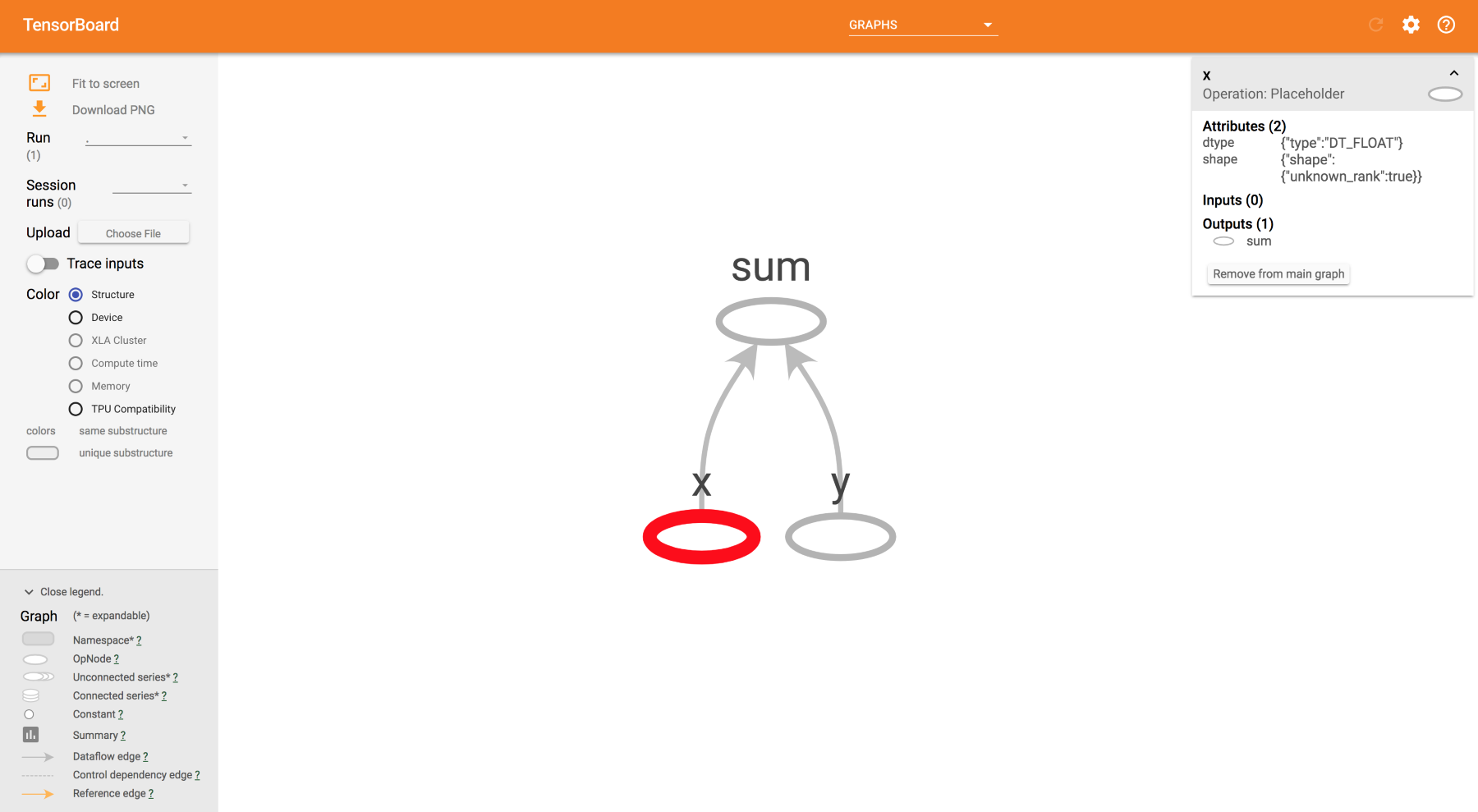The TensorBoard visualization in the browser window

The graph of addition is displayed with the names given for the placeholders. When we click on them, we can see all the particulars of the tensor for that operation on the right side. Make yourself familiar with the tabs and options. There are several parts in this window. We will learn about them in different chapters. TensorBoard is one the best distinguishing tools in TensorFlow, which makes it better than any other deep learning framework.

##### The TensorFlow Serving tool

TensorFlow Serving is a tool in TensorFlow developed for deployment environments that are flexible, providing high latency and throughput environments. Any deep learning model trained with TensorFlow can be deployed with serving. Install the Serving by running the following command:

`sudo apt-get install tensorflow-model-server`

Step-by-step instructions on how to use serving will be described in Chapter 3, Image Retrieval. Note that the Serving is easy to install only in Ubuntu; for other OSes, please refer to https://www.tensorflow.org/serving/setup. The following figure illustrates how TensorFlow Serving and TensorFlow interact in production environments: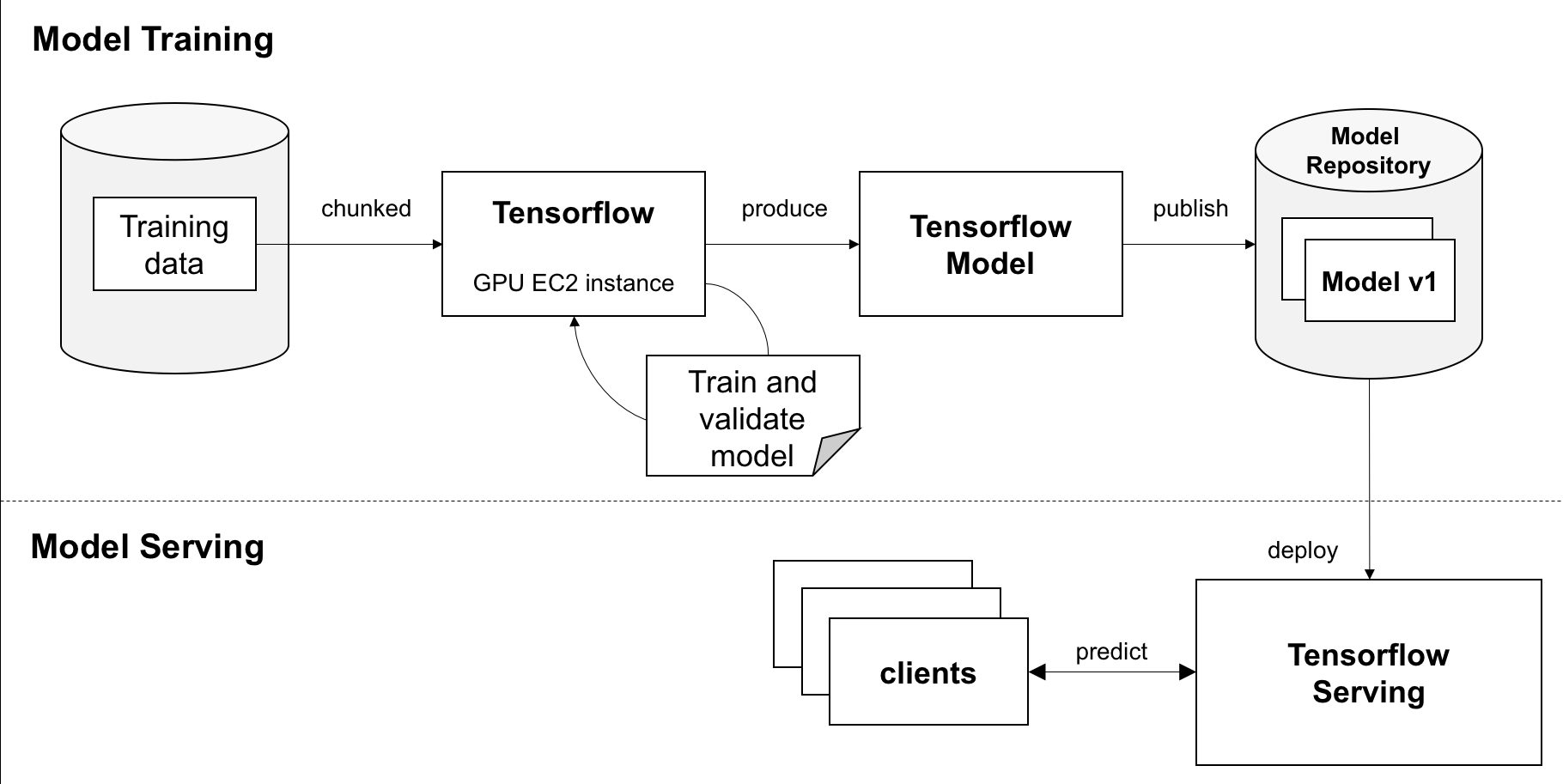Many models can be produced by the training process, and Serving takes care of switching them seamlessly without any downtime. TensorFlow Serving is not required for all the following chapters, except for Chapter 3, Image Retrieval and Chapter 10, Deployment.

#### The Keras library

`Keras` is an open source library for deep learning written in Python. It provides an easy interface to use TensorFlow as a backend. Keras can also be used with Theano, deep learning 4j, or CNTK as its backend. Keras is designed for easy and fast experimentation by focusing on friendliness, modularity, and extensibility. It is a self-contained framework and runs seamlessly between CPU and GPU. Keras can be installed separately or used within TensorFlow itself using the `tf.keras` API. In this book, we will use the `tf.keras` API. We have seen the steps to install the required libraries for the development environment. Having CUDA, CUDNN, OpenCV, TensorFlow, and Keras installed and running smoothly is vital for the following chapters.

## Summary

In this chapter, we have covered the basics of deep learning. The vocabulary introduced in this chapter will be used throughout this book, hence, you can refer back to this chapter often. The applications of computer vision are also shown with examples. Installations of all the software packages for various platforms for the development environment were also covered.

In the next chapter, we will discuss how to train classification models using both Keras and TensorFlow on a dataset. We will look at how to improve the accuracy using a bigger model and other techniques such as augmentation, and fine-tuning. Then, we will see several advanced models proposed by several people around the world, achieving the best accuracy in competitions.